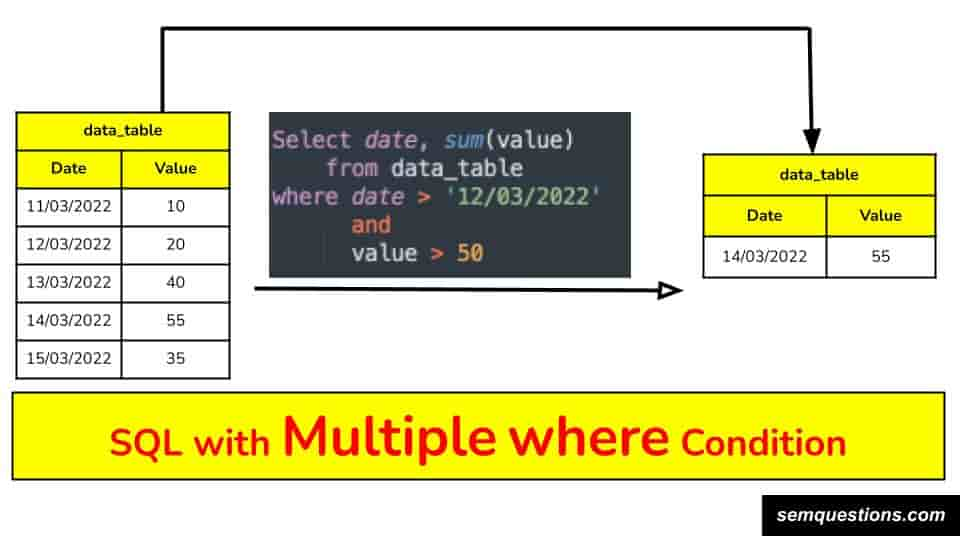# SQL Queries with Multiple where condition | SQL मे multiple where conditions कैसे डाले? – Data Analysis Tutorial

## Brief:

The SQL tutorial explains how to apply multiple where condition while writing SQL query, where the columns can be of type numerical, text or date.

There is restriction while applying this where clause i.e. it can be applied on:

• Two numerical columns,
• One numerical and One text column,
• One date and one text column,
• Two date columns and so on.

Reason why this function is important is it allows very selective filtering of data i.e. if you want to download data of India and only those rows where year should be less than 1998, or any other things like that then where clause makes it possible.

So, the function is not new, it’s been in use since a long time.

In future also this will be required so maybe some modification will happen to this function, but overall the selective filtering application of this function will be of use always.

## Why to rely on SQL multiple where conditions?

SQL where condition allow selective filtering of data as per the need.

Also, as you know dataset is never straight forward or easy to understand and sometimes it’s too big to even export on Google Sheet or excel for manual analysis reason being:

• Google Sheet and excel has limit upto which they can handle the data.
• Manual analysis is slow and is not error proof.
• Files becomes slow as data size increases.
• Manual processes takes time, whereas same SQL query can be used again and again etc.

So, because of above mentioned reasons SQL where condition function is important.

## Benefits of SQL queries with multiple where condition|sql मे multiple where conditions के फ़ायदे:

SQL queries with multiple where condition has advantages like easy analysis, time saving, automation of whole data fetching using tableau etc.

• Analysis becomes easy as all conditions can be applied using multiple where conditions and so there is no need to do any further analysis on excel or googlesheet.
• Saves time for future as same query can be used again and again.
• If someone has tableau then using the query one can create good visualisations and can put everything on automated refresh.

## Example 1: Applying SQL where clause on numerical columns.

SQL Query to filter data based on multiple conditions both on numerical column. Below example shows the visual presentation of the same.

So, in above example only those rows came out where salary is less than 25 and age is less 40 i.e. Rose and Theo in above case.

SQL query used is:

``Select * from data_table where salary < 25 and age < 40;``

Likewise you can put multiple condition on same column as well like

• salary < 25 and salary > 10, or
• age < 40 and age > 20 etc.

## Example 2: Applying SQL Where clause on one numerical and one text column.

In first example both the conditions were applied on numerical column, in this 2nd example one condition is applied on text column and another on numerical column.SQL with multiple where clause with 1st condition is on numerical column and another on text column.

So, in above example we are trying to get subject wise sum of weightage with subject equals to Math.

SQL query used is:

``Select subject, sum(weightage) from data_table where subject = 'Math' and weightage < 60;``

One exact use of this type of where condition can be used in cases where we want to check sales of same product on different dates and dates when sale is less than any particular threshold.

## Example 3: Applying SQL where clause on one date and one numerical column.

In this example one condition is applied on date column and another on numerical column.SQL with multiple where clause with 1st condition is on date column and another on numerical column.

Here, we are trying to find out sum of value column after 12/03/2022 date wise.

So, as we can see there are 3 rows with date > 12/03/2022 and only one row has value greater than 50, hence the result is only one row.

SQL query used is:

``Select date, sum(value) from data_table where date > '12/03/2022' and value > 50;``

Use case of such query can be in places where date is greater than x and sum of sales is greater than y, so you will get all those dates when the sale was greater than y.

## Example 4: Applying SQL where clause on two Date columns simultaneously.

In this example both condition is applied on date column.

Here, we are trying to find out sum of value column where start_date > ’12/03/2022′ and end_date < ’22/03/2022′, hence overall last 2 columns are qualified.

SQl query used is:

``Select sum(value) from data_Table where start_Date > '12/03/2022' and end_Date < '22/03/2022';``

One use case of such application of where clause on date column is finding out all the sales between x start date and y end date.

## Using where clause with REGEX (e.g. like function):

SQL where clause is not restricted only to exact or comparative matching of numerical, text, dates etc.

We can use regex in it and can do approximate matching as well for e.g.

select * from data where column_a > 20 and name like ‘%kia%’;

The above example will return all the rows where value of column_a is greater than 20 and name column has keyword kia in it.

So, all the rows will have keyword kia in the name column.

Reason why this is important is because sometimes in big datasets we don’t know exact values that we want and we just have some logic to get the data, so in such cases regex is veryhelpful.